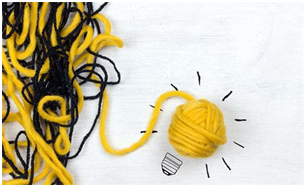①东晴是18岁。

②印玉去了A岛。

21岁的女孩子发现的蛋的数量比去A岛女孩的多1个。

19岁的女孩子发现的蛋的数量比去B岛女孩的多1个。

⑤张虹发现的蛋和C岛的蛋之中，有一者是2个。

D岛的蛋比西雨的蛋要多2个。

315*8-1=2519 11|2519 所以最少有2519 2519/11=229() 所以屋子里有229张桌子 答：这间屋子有2519个人，有229张桌子。

315 - 1 = 314 314÷11=28.5455 (除不尽保留前四位小数)

315×2-1=629 629÷11=57.1818 315×3-1=944

944÷11=85.8182 … … … … … … … …

315×8-1=2519 2519÷11=229 (第一个符合题目要求的数) 所以答案应该是 2519个人 ,当然这个屋子就必须很大咯才能容得下这么多人

3个人(3个人=3×2=6条腿)

1匹马加27只大猫加81只小猫加243只小猫带的老鼠加81只大猫带的老鼠等于433433乘以4等于1732条腿。加4个人8条腿。共1740条腿。

2只腿的人的数量为1+3=4

4+8+144+432+1296=1884条腿

158个小朋友。10个小朋友拿到梨和苹果最少人数是(2+1)×(4+1)×(101)+1=136人，然后从左右两端开始向外延伸，假设梨和苹果都拿到的人为“1”，左右两边的延伸数分别为：3×5-3=12人，3×5-5=10人。所以，总人数为136+12+10=158